Courses

# Past Year Questions: Hydrostatic Forces Notes | EduRev

## Civil Engineering (CE) : Past Year Questions: Hydrostatic Forces Notes | EduRev

The document Past Year Questions: Hydrostatic Forces Notes | EduRev is a part of the Civil Engineering (CE) Course Fluid Mechanics.
All you need of Civil Engineering (CE) at this link: Civil Engineering (CE)

Question 1. A sector gate is provided on a spillway as shown in the figure. Assuming g = 10 m/s2, the resultant force per meter length (expressed in kN/m) on the gate will be_______ .     [2016 : 2 Marks, Set-I]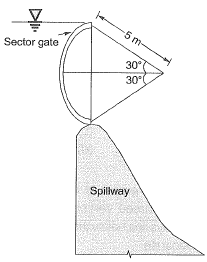Solution: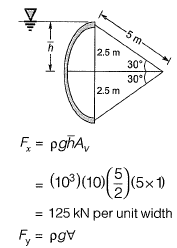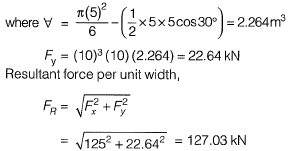Question 2. A triangular gate with a base width of 2 m and a height of 1.5 m lies in a vertical plane. The top vertex of the gate is 1.5 m below the surface of a tank which contains oil of specific gravity 0.8. Considering the density of water and acceleration due to gravity to be 1000 kg/m3 and 9.81 m/s2, respectively, the hydrostatic force (in kN) exerted by the oil on the gate is _________ .     [2015 : 2 Marks, Set-II]
Solution: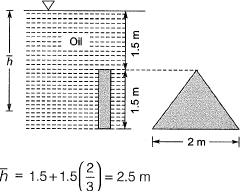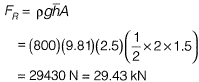Question 3. Three rigid buckets, shown as in the figures (1), (2) and (3), are of identical heights and base areas. Further, assume that each of these buckets have negligible mass and are full of water. The weight of water in these buckets are denoted as W1,  W2, and W3 respectively. Also, let the force of water on the base of the bucket be denoted as F1, F2, and F3 respectively. The option giving an accurate description of the system physics is    [2014 : 2 Marks, Set-I]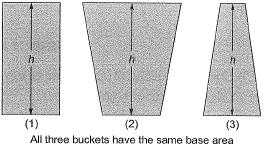(a) W2 = W1 = W3 and F2 > F1 > F
(b) W2 > W1 > W3 and F2 > F1 > F
(c) W2 = W1 = W3 and F1 = F2 = F
(d) W2 > W1 > W3 and F1 = F2 = F
(d)
Solution:
Bucket → identical height → identical base area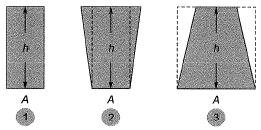=> w2> w1> w
Force on the base in each case will be equal to =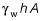Hence, F1 = P2 = F3

Offer running on EduRev: Apply code STAYHOME200 to get INR 200 off on our premium plan EduRev Infinity!

## Fluid Mechanics

43 videos|70 docs|72 tests

,

,

,

,

,

,

,

,

,

,

,

,

,

,

,

,

,

,

,

,

,

;